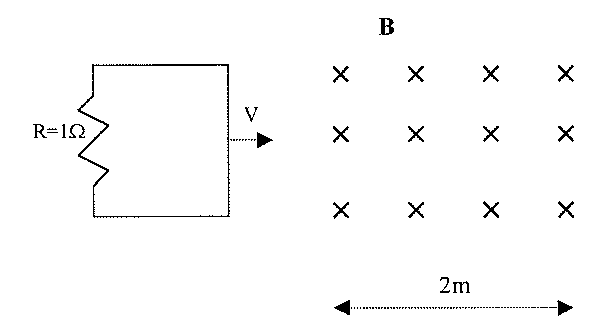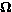## University of California, San Diego Physics 1b - Thermal Physics & Electromagnetism

 H. E. Smith Spring 2000Physics 1B - Tutorial #101. The figure above shows two coils, A & B. Use Lenz' Law to determine which direction current flows through the resistor when
• (a) the switch is closed.
• (b) the switch is opened after being closed.
• (c) the current in A decreases.
• (d) the current in A increases.
• (e) B is moved toward A.2. The conducting loop shown above has area A = 0.01m2 and is moving with v=1m/s into a magnetic field B = 10-4T. Graph the current in the loop as a function of time.

3. An apartment complex is supplied by single-phase AC from a power plant 5-km away. The wire that runs from the power plant to the complex and back has a resistance per unit length of 1 x 10-5/m, supplying 100,000W.
• Calculate the resistive power losses in the wire if the AC is supplied at 110V.
• Calculate the resistive power losses in the wire if the AC is supplied at 500kV.
• Design a way to transform AC provided at 500kV to 110V.Physics 1B HomeTutorials

Gene Smith